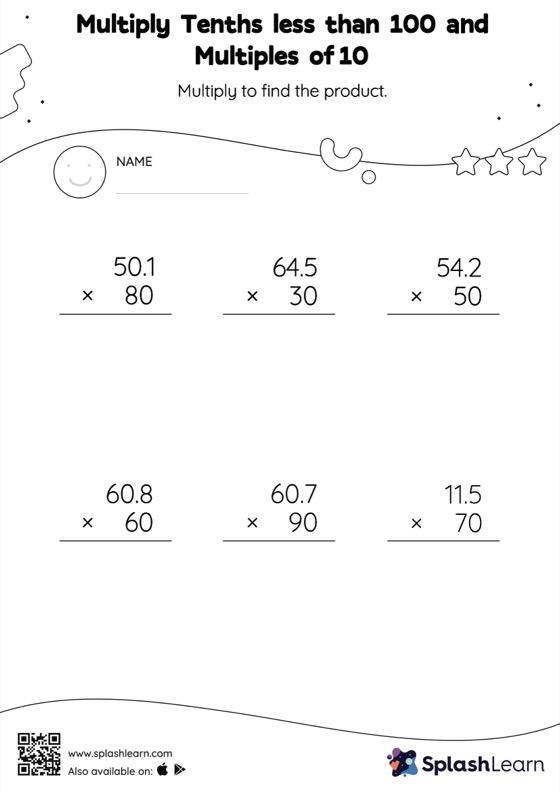# Multiply Tenths less than 100 and Multiples of 10: Vertical Multiplication Worksheet

Home > Multiply Tenths less than 100 and Multiples of 10: Vertical MultiplicationGive your little one some practice building multiplication skills with this multiply tenths less than 100 and multiples of 10 worksheet. Students understand that multiplying a decimal by a number that ends in zeros causes the decimal point to be placed in the product as many places from the right as the number of decimal digits in the multiplicand. In multiply tenths less than 100 and multiples of 10 worksheet, they practice this idea in great detail.